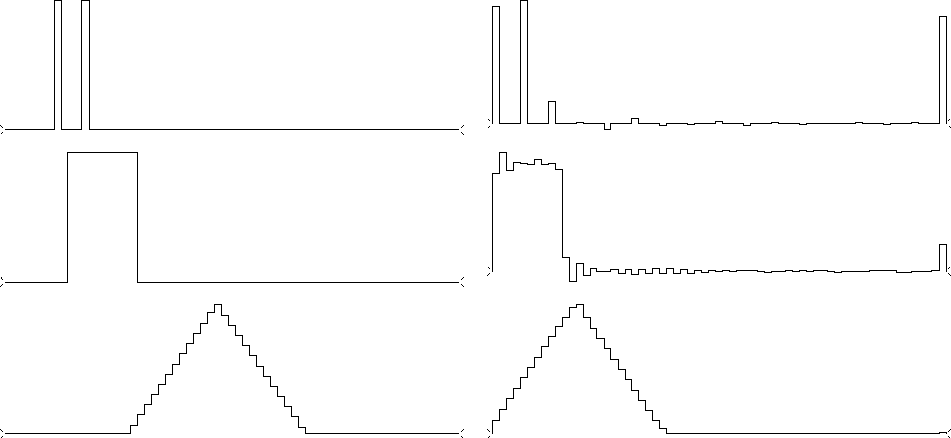Next: Relation of amplitude to Up: SPECTRAL FACTORIZATION Previous: Why the causal wavelet

## Pathological examples

The spectral-factorization algorithm fails when an infinity is encountered. This happens when the spectrum becomes zero, so that its logarithm becomes minus infinity. This can occur in a benign way--for example, in the case of the spectrum of the wavelet (1,1), where the infinity occurs at the Nyquist frequency. We could smooth the spectrum near the Nyquist before we take the logarithm. On the other hand, the pathology can be more extreme. Convolving (1,1) with itself N times, we see that the result and its spectrum tend to Gaussians. So, at the Nyquist frequency, smoothing would only replace zero by a very tiny number.

Figure 12 shows functions whose spectra contain zeros, along with their minimum-phase equivalents. When the logarithm of zero arises during the computation, it is replaced by the log of 10-30. It is surprising that the triangle suffered so much less than the other two functions. It seems that minor imperfection in specifying the triangle resulted in a spectrum that did not have the theoretical zeros of sinc squared.

 patho Figure 12 Functions whose spectra contain zeros, along with their minimum-phase equivalents, as computed by discrete Fourier transform.Next: Relation of amplitude to Up: SPECTRAL FACTORIZATION Previous: Why the causal wavelet
Stanford Exploration Project
10/21/1998